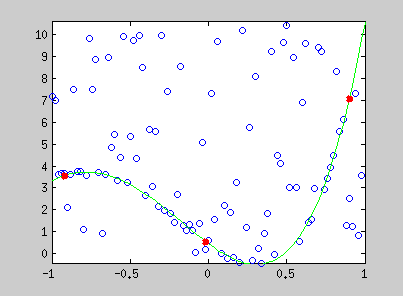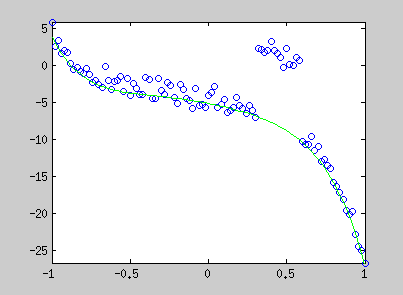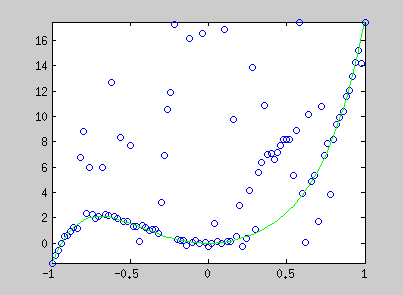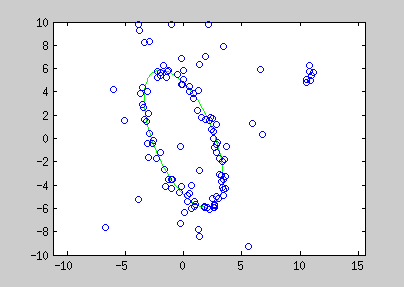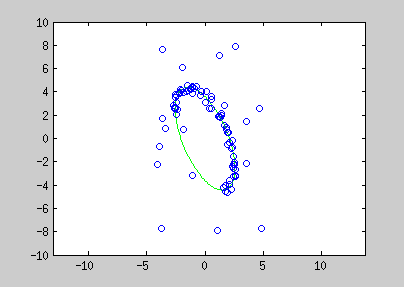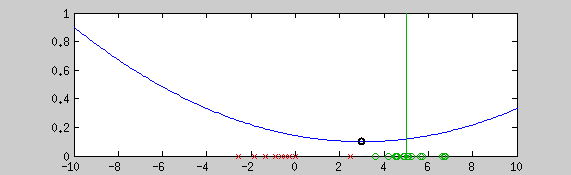This example shows the estimation of the mean with the graduate non convexity approach using a newton optimization at each step for locally minimizing the energy. Green points are a realization of a normal distribution (vertical line shows the mean), red crosses are outliers. The blue line is the normalized cost function. The algorithm starts from a convex estimator (the normal distribution in this case). Step by step, a more robust estimator is taken. The black point shows the current estimate of the mean.

### Introduction

Many problems have a non convex energy formulation. Therefore, reaching the global minimal in a finite time is not guaranteed. Non convex functions are often used for robust estimation to estimate variable under non-Gaussian noise. The graduated non convexity approach provides a generic method for reaching an interesting minima when robust estimators are needed.

### Generic approach for robust linear fitting

For many problems, we are looking for model parameters $$\phi$$ of a probability function which maximize the given function from paired training examples $$y_i$$ and $$x_i$$ :

${\underset{\mathrm{\phi}}{\text{argmax}}}\;[ Pr(y_i | x_i,\phi) ]$

Where the variable $$y_i$$ is linearly related to $$x_i$$ by $$\phi$$ as following :

$y_i = \phi x_i + \epsilon$

$$\epsilon$$ is the perturbation related to the noise distribution. When the noise is supposed gaussian, the normal distribution is chosen and this leads to the well known least square problem when the negative-log of the pdf is taken. This formulation is very sensitive to outliers because of the Gaussian noise assumption. To tackle this problem, one will choose a robust estimator instead of the normal distribution.